UIHistories Project: A History of the University of Illinois by Kalev Leetaru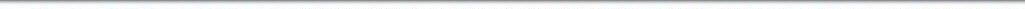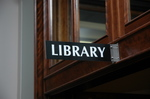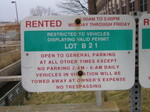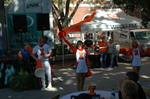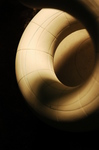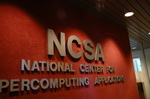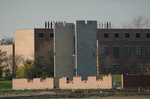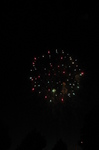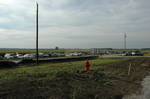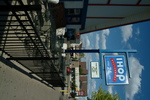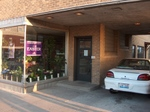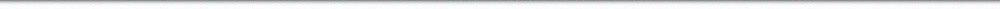N A V I G A T I O N D I G I T A L L I B R A R Y

## Repository: UIHistories Project: Board of Trustees Minutes - 1896 [PAGE 122]

Caption: Board of Trustees Minutes - 1896
This is a reduced-resolution page image for fast online browsing.

< Previous Page [Displaying Page 122 of 371] Next Page >
[VIEW ALL PAGE THUMBNAILS]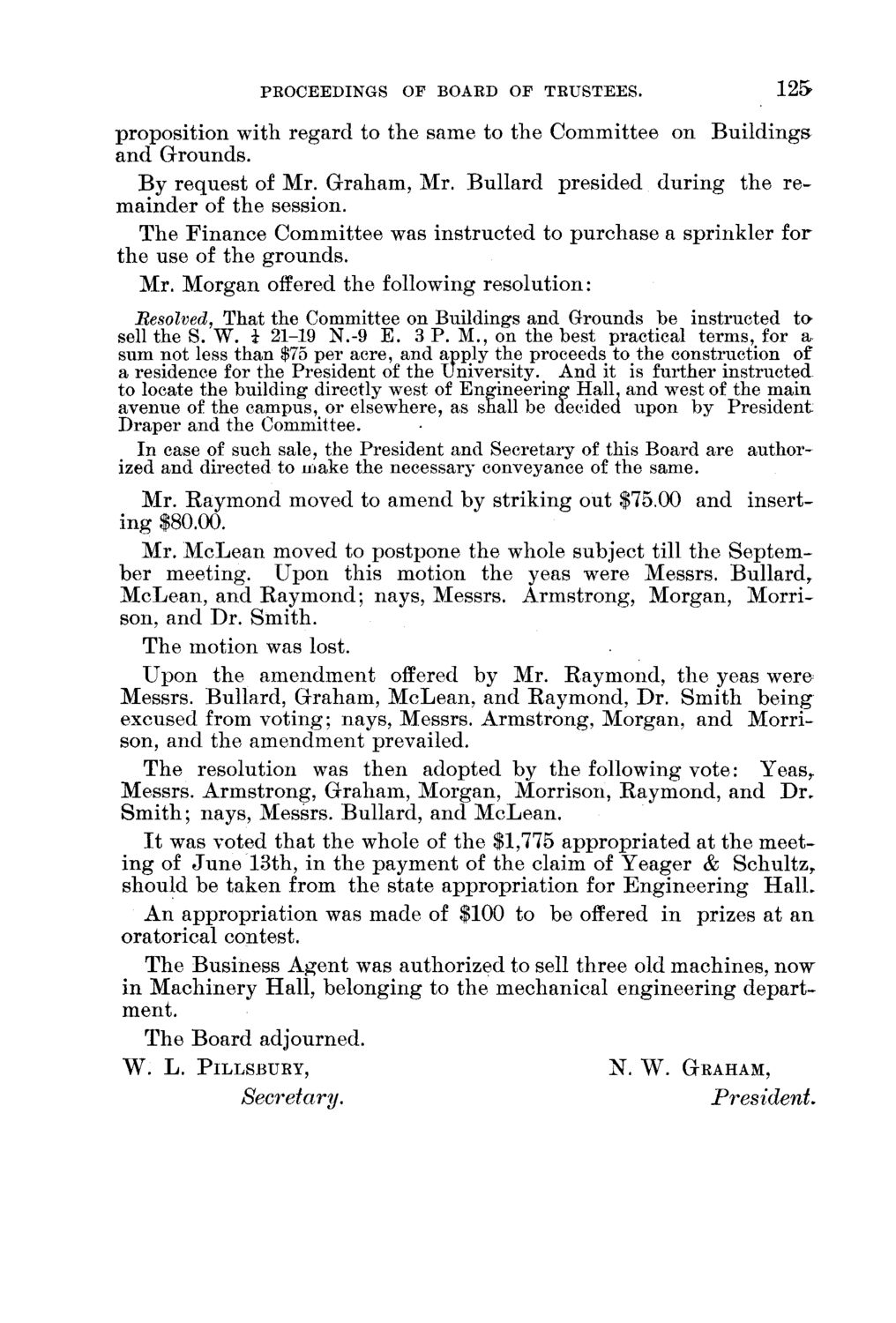### EXTRACTED TEXT FROM PAGE:

PEOCEEDINGS OF BOAED OF TRUSTEES.

125

p r o p o s i t i o n with r e g a r d to t h e same to t h e C o m m i t t e e on B u i l d i n g s and Grounds. B y r e q u e s t of Mr. G r a h a m , Mr. B u l l a r d p r e s i d e d d u r i n g t h e r e m a i n d e r of t h e session. T h e F i n a n c e C o m m i t t e e was i n s t r u c t e d to p u r c h a s e a s p r i n k l e r for t h e use of t h e g r o u n d s . Mr. M o r g a n offered t h e following r e s o l u t i o n : Besolved, That the Committee on Buildings and Grounds be instructed t a sell the S. W. i 21-19 N.-9 E. 3 P. M., on the best practical terms, for a, sum not less than \$75 per acre, and apply the proceeds to the construction of a residence for the President of the university. And it is further instructed to locate the building directly west of Engineering Hall, and west of the main avenue of the campus, or elsewhere, as shall be decided upon by President Draper and the Committee. In case of such sale, the President and Secretary of this Board are authorized and directed to make the necessary conveyance of the same. Mr. R a y m o n d moved to a m e n d by s t r i k i n g out \$75.00 a n d i n s e r t i n g \$80.00. Mr. M c L e a n moved to p o s t p o n e t h e whole subject till t h e S e p t e m b e r m e e t i n g . U p o n t h i s motion t h e yeas were Messrs. B u l l a r d , M c L e a n , a n d R a y m o n d ; nays, Messrs. A r m s t r o n g , M o r g a n , M o r r i son, and D r . S m i t h . T h e motion was lost. U p o n t h e a m e n d m e n t offered by Mr. R a y m o n d , t h e yeas were Messrs. Bullard, G r a h a m , M c L e a n , a n d R a y m o n d , D r . S m i t h b e i n g excused from v o t i n g ; nays, Messrs. A r m s t r o n g , M o r g a n , a n d M o r r i son, a n d t h e a m e n d m e n t prevailed. T h e resolution was t h e n a d o p t e d by t h e following v o t e : Y e a s r Messrs. A r m s t r o n g , G r a h a m , M o r g a n , Morrison, R a y m o n d , a n d D r . S m i t h ; nays, Messrs. B u l l a r d , a n d M c L e a n . I t was voted t h a t t h e whole of t h e \$1,775 a p p r o p r i a t e d at t h e m e e t i n g of J u n e 13th, in t h e p a y m e n t of t h e claim of Y e a g e r & Schultz y should be t a k e n from t h e state a p p r o p r i a t i o n for E n g i n e e r i n g H a l L A n a p p r o p r i a t i o n was m a d e of \$100 to be offered in prizes at a n oratorical contest. T h e B u s i n e s s A g e n t was a u t h o r i z e d to sell t h r e e old m a c h i n e s , n o w i n M a c h i n e r y H a l l , b e l o n g i n g to t h e m e c h a n i c a l e n g i n e e r i n g d e p a r t ment. T h e B o a r d adjourned.

W. L. PILLSBURY, N. W. GRAHAM,

Secretary.

President,